1624229160

# K-Nearest Neighbors, Clearly Explained

Machine learning and Data Mining sure sound like complicated things, but that isn’t always the case. Here we talk about the surprisingly simple and surprisingly effective K-nearest neighbors algorithm.

#machine-learning

## Buddha Community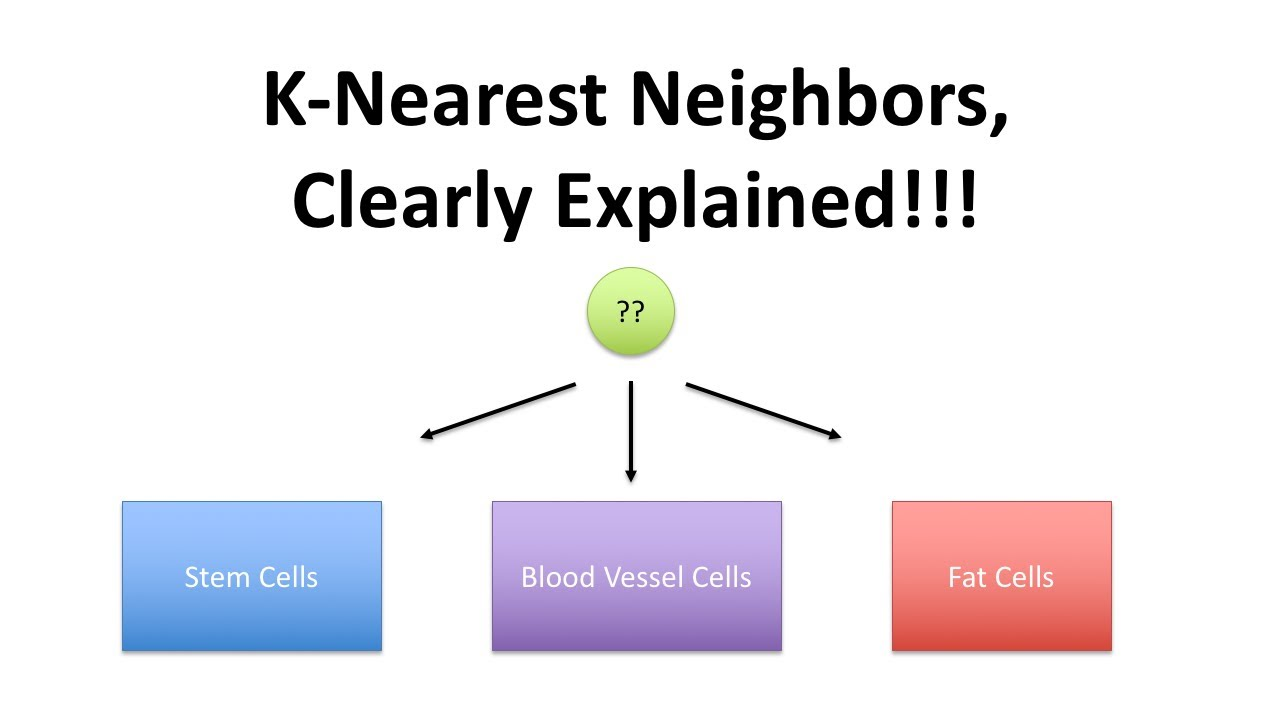1596905700

## K-Nearest Neighbors Algorithm

KNN is a non-parametric and lazy learning algorithm. Non-parametric means there is no assumption for underlying data distribution. In other words, the model structure determined from the dataset. This will be very helpful in practice where most of the real-world datasets do not follow mathematical theoretical assumptions.

KNN is one of the most simple and traditional non-parametric techniques to classify samples. Given an input vector, KNN calculates the approximate distances between the vectors and then assign the points which are not yet labeled to the class of its K-nearest neighbors.

The lazy algorithm means it does not need any training data points for model generation. All training data used in the testing phase. This makes training faster and the testing phase slower and costlier. The costly testing phase means time and memory. In the worst case, KNN needs more time to scan all data points, and scanning all data points will require more memory for storing training data.

## K-NN for classification

Classification is a type of supervised learning. It specifies the class to which data elements belong to and is best used when the output has finite and discrete values. It predicts a class for an input variable as well.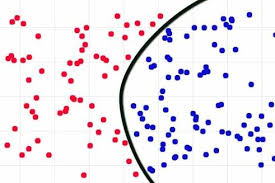Consider given review is positive (or) Negative, classification is all about if we give a new query points determine (or) predict the given review is positive (or) Negative.

Classification is all about learning the function for given points.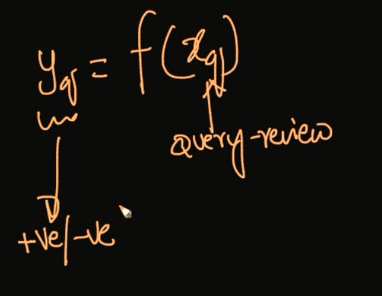How does the K-NN algorithm work?

In K-NN, K is the number of nearest neighbors. The number of neighbors is the core deciding factor. K is generally an odd number if the number of classes is 2. When K=1, then the algorithm is known as the nearest neighbor algorithm. This is the simplest case. Suppose P1 is the point, for which label needs to predict. First, you find the one closest point to P1 and then the label of the nearest point assigned to P1.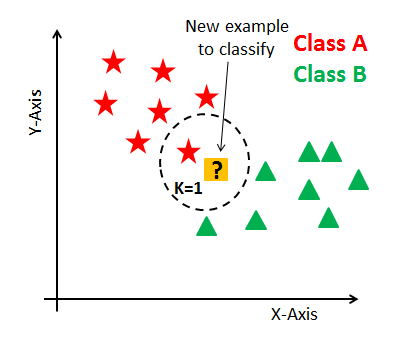Suppose P1 is the point, for which label needs to predict. First, you find the k closest point to P1 and then classify points by majority vote of its k neighbors. Each object votes for their class and the class with the most votes is taken as the prediction. For finding closest similar points, you find the distance between points using distance measures such as Euclidean distance, Hamming distance, Manhattan distance, and Minkowski distance.

K-NN has the following basic steps:

1. Calculate distance
2. Find closest neighbors
3. Vote for labels
4. Take the majority Vote

Failure Cases of K-NN:

_1.When Query Point is far away from the data points.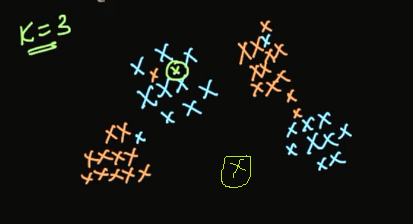2.If we have Jumble data sets.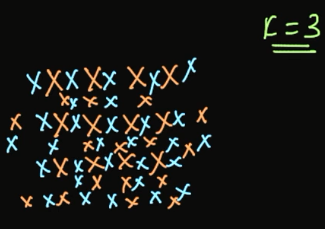For the above image shows jumble sets of data set, no useful information in the above data set. In this situation, the algorithm may be failing.

_Distance Measures in K-NN: _There are mainly four distance measures in Machine Learning Listed below.

1. Euclidean Distance
2. Manhattan Distance
3. Minkowski Distance
4. Hamming Distance

Euclidean Distance

The Euclidean distance between two points in either the plane or 3-dimensional space measures the length of a segment connecting the two points. It is the most obvious way of representing distance between two points. Euclidean distance marks the shortest route of the two points.

The Pythagorean Theorem can be used to calculate the distance between two points, as shown in the figure below. If the points (x1,y1)(x1,y1) and (x2,y2)(x2,y2) are in 2-dimensional space, then the Euclidean distance between them is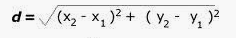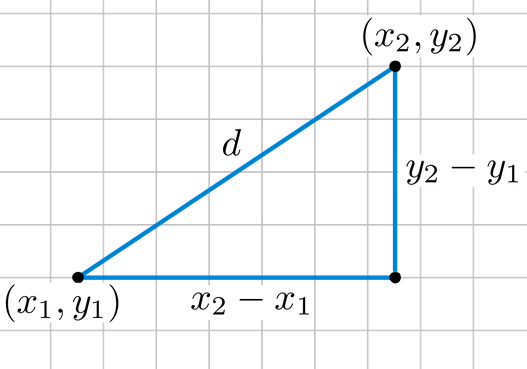Euclidean distance is called an L2 Norm of a vector.

Norm means the distance between two vectors.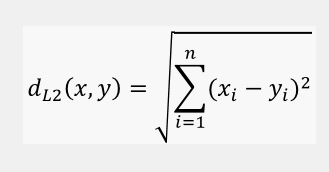Euclidean distance from an origin is given by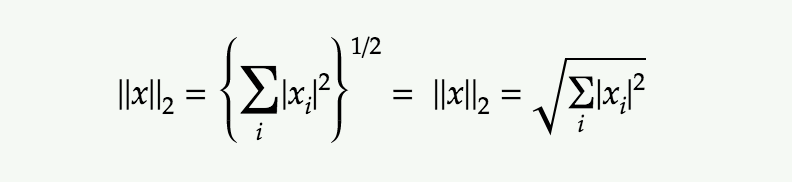Manhattan Distance

The Manhattan distance between two vectors (city blocks) is equal to the one-norm of the distance between the vectors. The distance function (also called a “metric”) involved is also called the “taxi cab” metric.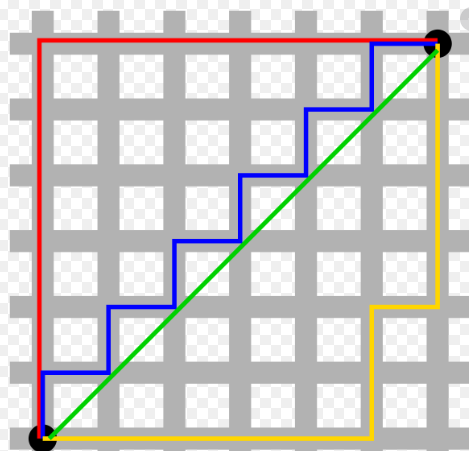Manhattan distance between two vectors is called as L1 Norm of a vector.

In L2 Norm we take the sum of the Squaring of the difference between elements vectors, in L1 Norm we take the sum of the absolute difference between elements vectors.

Manhattan Distance between two points (x1, y1) and (x2, y2) is:

|x1 — x2| + |y1 — y2|.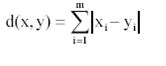Manhattan Distance****from an origin is given by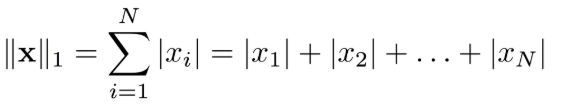Minkowski Distance

Minkowski distance__is a metric in a normed vector space. Minkowski distance is used for distance similarity of vector. Given two or more vectors, find distance similarity of these vectors.

#analytics #machine-learning #applied-ai #data-science #k-nearest-neighbors #data analytic1602745200

## Exploring The Brute Force K-Nearest Neighbors Algorithm

Did you find any difference between the two graphs?

Both show the accuracy of a classification problem for K values between 1 to 10.

Both of the graphs use the KNN classifier model with ‘Brute-force’ algorithm and ‘Euclidean’ distance metric on same dataset. Then why is there a difference in the accuracy between the two graphs?

Before answering that question, let me just walk you through the KNN algorithm pseudo code.

I hope all are familiar with k-nearest neighbour algorithm. If not, you can read the basics about it at https://www.analyticsvidhya.com/blog/2018/03/introduction-k-neighbours-algorithm-clustering/.

We can implement a KNN model by following the below steps:

2. Initialise the value of k
3. For getting the predicted class, iterate from 1 to total number of training data points
4. Calculate the distance between test data and each row of training data. Here we will use Euclidean distance as our distance metric since it’s the most popular method. Some of the other metrics that can be used are Chebyshev, cosine, etc.
5. Sort the calculated distances in ascending order based on distance values
6. Get top k rows from the sorted array
7. Get the most frequent class of these rows
8. Return the predicted class

#2020 oct tutorials # overviews #algorithms #k-nearest neighbors #machine learning #python1599448320

## Under the Hood of K-Nearest Neighbors (KNN) and Popular Model Validation Techniques

This article contains in-depth algorithm overviews of the K-Nearest Neighbors algorithm (Classification and Regression) as well as the following Model Validation techniques: Traditional Train/Test Split and Repeated K-Fold Cross Validation. The algorithm overviews include detailed descriptions of the methodologies and mathematics that occur internally with accompanying concrete examples. Also included are custom, fully functional/flexible frameworks of the above algorithms built from scratch using primarily NumPy. Finally, there is a fully integrated Case Study which deploys several of the custom frameworks (KNN-Classification, Repeated K-Fold Cross Validation) through a full Machine Learning workflow alongside the Iris Flowers dataset to find the optimal KNN model.

Please use the imports below to run any included code within your own notebook or coding environment.

``````import pandas as pd
import numpy as np
import matplotlib.pyplot as plt
import seaborn as sns
from statistics import mean,stdev
from itertools import combinations
import math
%matplotlib inline
``````

### KNN Algorithm Overview

K-Nearest Neighbors is a popular pattern recognition algorithm used in Supervised Machine Learning to handle both classification and regression-based tasks. At a high level, this algorithm operates according to an intuitive methodology:

New points target values are predicted according to the target values of the K most similar points stored in the model’s training data.

Traditionally, ‘similar’ is interpreted as some form of a distance calculation. Therefore, another way to interpret the KNN prediction methodology is that predictions are based off the K closest points within the training data, hence the name K-Nearest Neighbors. With the concept of distance introduced, a good initial question to answer is how distance will be computed. While there are several different mathematical metrics that are viewed as a form of computing distance, this study will highlight the 3 following distance metrics: Euclidean, Manhattan, and Chebyshev.

### KNN Algorithm Overview — Distance Metrics

Euclidean distance, based in the Pythagorean Theorem, finds the straight line distance between two points in space. In other words, this is equivalent to finding the shortest distance between two points by drawing a single line between Point A and Point B. Manhattan distance, based in taxicab geometry, is the sum of all N distances between Point A and Point B in N dimensional feature space. For example, in 2D space the Manhattan distance between Point A and Point B would be the sum of the vertical and horizontal distance. Chebyshev distance is the maximum distance between Point A and Point B in N dimensional feature space. For example, in 2D space the Chebyshev distance between Point A and Point B would be max(horizontal distance, vertical distance), in other words whichever distance is greater between the two distances.

Consider Point A = (A_1, A_2, … , A_N) and Point B = (B_1, B_2, … , B_N) both exist in N dimensional feature space. The distance between these two points can be described by the following formulas: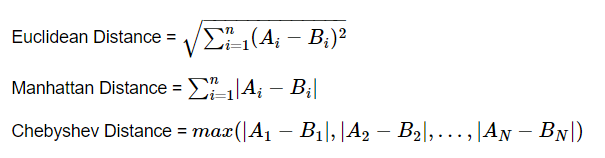As with most mathematical concepts, distance metrics are often easier to understand with a concrete example to visualize. Consider Point A = (0,0) and Point B = (3,4) in 2D feature space.

``````sns.set_style('darkgrid')
fig = plt.figure()
axes.scatter(x=[0,3],y=[0,4],s = 50)
axes.plot([0,3],[0,4],c='blue')
axes.annotate('X',[1.5,1.8],fontsize = 14,fontweight = 'bold')
axes.plot([0,0],[0,4],c='blue')
axes.annotate('Y',[-0.1,1.8],fontsize = 14,fontweight = 'bold')
axes.plot([0,3],[4,4],c='blue')
axes.annotate('Z',[1.5,4.1],fontsize = 14,fontweight = 'bold')
axes.annotate('(0,0)',[0.1,0.0],fontsize = 14,fontweight = 'bold')
axes.annotate('(3,4)',[2.85,3.7],fontsize = 14,fontweight = 'bold')
axes.grid(lw=1)
plt.show()
``````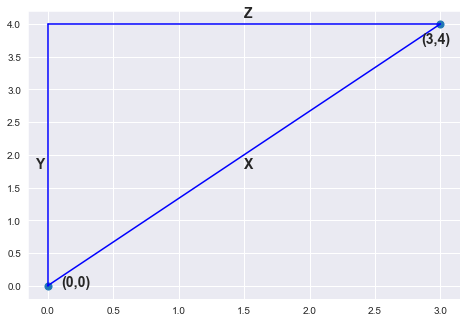#python #machine-learning #siri #k-nearest-neighbors #crossvalidation1593571140

## K-Nearest Neighbors

A perfect opening line I must say for presenting the K-Nearest Neighbors. Yes, that’s how simple the concept behind KNN is. It just classifies a data point based on its few nearest neighbors. How many neighbors? That is what we decide.

Looks like you already know a lot of there is to know about this simple model. Let’s dive in to have a much closer look.

Before moving on, it’s important to know that KNN can be used for both classification and regression problems. We will first understand how it works for a classification problem, thereby making it easier to visualize regression.

## KNN Classifier

The data we are going to use is the Breast Cancer Wisconsin(Diagnostic) Data Set_. _There are 30 attributes that correspond to the real-valued features computed for a cell nucleus under consideration. A total of 569 such samples are present in this data, out of which 357 are classified as ‘benign’ (harmless) and the rest 212 are classified as _‘malignant’ _(harmful).

The diagnosis column contains ‘M’ or ‘B’ values for malignant and benign cancers respectively. I have changed these values to 1 and 0 respectively, for better analysis.

Also, for the sake of this post, I will only use two attributes from the data → ‘mean radius’ and ‘mean texture’. This will later help us visualize the decision boundaries drawn by KNN. Here’s how the final data looks like (after shuffling):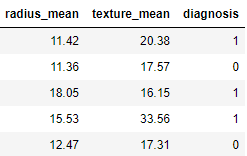Let’s code the KNN:

``````# Defining X and y
X = data.drop('diagnosis',axis=1)
y = data.diagnosis

# Splitting data into train and test
from sklearn.model_selection import train_test_split
X_train, X_test, y_train, y_test = train_test_split(X,y,test_size=0.25,random_state=42)
# Importing and fitting KNN classifier for k=3
from sklearn.neighbors import KNeighborsClassifier
knn = KNeighborsClassifier(n_neighbors=3)
knn.fit(X_train,y_train)
# Predicting results using Test data set
pred = knn.predict(X_test)
from sklearn.metrics import accuracy_score
accuracy_score(pred,y_test)
``````

The above code should give you the following output with a slight variation.

``````0.8601398601398601
``````

What just happened? When we trained the KNN on training data, it took the following steps for each data sample:

1. Calculate the distance between the data sample and every other sample with the help of a method such as Euclidean.
2. Sort these values of distances in ascending order.
3. Choose the top K values from the sorted distances.
4. Assign the class to the sample based on the most frequent class in the above K values.

Let’s visualize how KNN drew a decision boundary on the train data set and how the same boundary is then used to classify the test data set.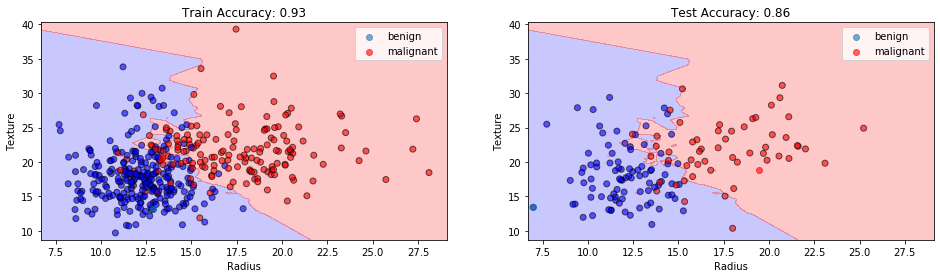KNN Classification at K=3. Image by Sangeet Aggarwal

With the training accuracy of 93% and the test accuracy of 86%, our model might have shown overfitting here. Why so?

When the value of K or the number of neighbors is too low, the model picks only the values that are closest to the data sample, thus forming a very complex decision boundary as shown above. Such a model fails to generalize well on the test data set, thereby showing poor results.

The problem can be solved by tuning the value of _n_neighbors _parameter. As we increase the number of neighbors, the model starts to generalize well, but increasing the value too much would again drop the performance.

Therefore, it’s important to find an optimal value of K, such that the model is able to classify well on the test data set. Let’s observe the train and test accuracies as we increase the number of neighbors.

#knn-algorithm #data-science #knn #nearest-neighbors #machine-learning #algorithms1592828700

## K Nearest Neighbors

KNN falls in the supervised learning family of algorithms. K nearest neighbors is a simple algorithm that stores all available cases and classifies new cases based on a similarity measure. Classification is done by a majority vote to its neighbors. The data is assigned to the class which has the most nearest neighbors. As you increase the number of nearest neighbors, the value of k, accuracy might increase.
KNN is a non-parametric, lazy learning algorithm. When we say a technique is non-parametric , it means that it does not make any assumptions on the underlying data distribution.

#data-science #machine-learning #python #k-nearest-neighbours #data-analysis